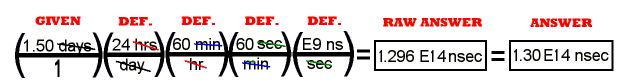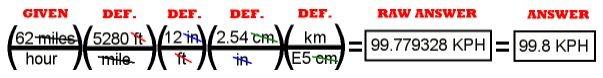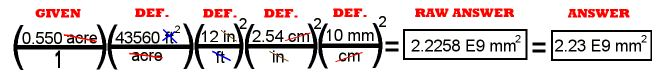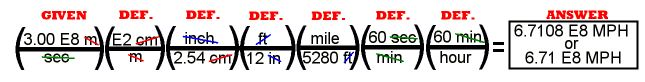PROBLEM #1, SET 1 – NUMBERS

1. What is 1.50 mm in km?

This problem is clearly a DA – only problem because it asks you to convert
from one unit of distance to another, mm to km. Both of the units are metric
units, so it needs a metric definiton.
Either use the common definitions of kilometer to meter and meter to millimeter
or use the metric staircase.
Notice in each of the definitions that one of the larger unit comes first.

1 km = E 6 mm
or
1 km = E 3 m and 1 m = E 3 mm

As for any DA problem, begin with what you know and use the definitions as conversion
factors so you can cancel the units you don’t want and leave the units you do.ORE6 is 1,000,000. The calculator math is:
1.5 ÷ 1 E 6 = or 1.5 ÷ 1000000 =

PROBLEM #2, SET 1 – NUMBERS

2. How many nanoseconds are in 1.50 days?

This problem is a DA – only problem because it asks you to convert from one unit
of time to another, nanoseconds to days. Seconds to nanoseconds is a metric definition.
See the metric staircase or the definition of "nano-." Seconds to days are commonly known definitions, seconds to minutes to hours to days.
Notice in each of the definitions that one of the larger unit comes first.

1 sec = E 9 nanoseconds
1 min = 60 seconds
1 hour = 60 min
1 day = 24 hours

As for any DA problem, begin with what you know and use the definitions as conversion
factors so you can cancel the units you don’t want and leave the units you do.The math is done by multiplying everything on top (in the numerator) and dividing by everything on the
bottom (in the denominator). It is best done with a calculator SEQUENTIALLY, in the following manner:

1.50 x 24 x 60 x 60 x 1 E 9 =

There is no need to notice intermediate answers or to write anything down until you
reach the end of the math. On most scientific calculators it is easiest to put in E9 by
first inserting a 1.

The raw answer is the number taken directly off of the calculator and the correct units. The final answer
is given here shortened to three significant figures. Your calculator will show you large numbers in the scientific notation style.

PROBLEM #3, SET 1 – NUMBERS

3. How many fluid ounces (floz.) of water do you have in exactly half of a ten gallon can?

This problem is a
DA – only problem beacuse it asks you to convert from one unit
of volume to another, gallons to fluid ounces. The definitions are all English.
Notice in each of the definitions that one of the larger unit comes first.

1 gal = 4 qt.
1 qt. = 2 pints
1pint = 2 cups
1cup = 8 floz.

As for any DA problem, begin with what you know and use the definitions as conversion
factors so you can cancel the units you don’t want and leave the units you do.
This example begins with "half of a ten gallon can," so there is a number in the denominator of "given."The math is done by multiplying everything on top (in the numerator) and dividing by everything on the
bottom (in the denominator). On the calculator that is:
10 ÷ 2 x 4 x 2 x 2 x 8 =

There is no need to notice intermediate answers or to write anything down until you
reach the end of the math.

PROBLEM #4, SET 1 – NUMBERS

4. A car is going 60.0 MPH. How fast is that in ft/sec?

This problem is a
DA – only problem beacuse it asks you to convert from one unit
of velocity to another, miles per hour to feet per second. The definitions are all English.
Notice in each of the definitions that one of the larger unit comes first.

1 mile = 5280 ft
1 hr = 60 min
1 min = 60 sec

Now is a good time to mention a common mistake. In changing hours to seconds I have seen many
students use "1 hr = 60 sec" as a conversion factor. That’s a mighty short hour.

As for any DA problem, begin with what you know and use the definitions as conversion
factors so you can cancel the units you don’t want and leave the units you do.
This example begins with "sixty miles per hour." "MPH" is just an abbreviation for
"miles per hour," and the "hour" rightly goes in the denominator. The "miles" must be changed
to "feet," and the "hours" must be changed to "seconds."The math is done by multiplying everything on top (in the numerator) and dividing by everything on the
bottom (in the denominator). If you do it the long way, the calculator math is:

60 x 5280 ÷ 60 ÷ 60 =
If you want to shorten it, cancel one of the "60’s" in the denominator with "60" in the numerator to make the
math:
5280 ÷ 60 =

The answer shows three significant digits by showing the zero after the decimal point, even though that zero does not appear on the calculator.

There is no need to notice intermediate answers or to write anything down until you
reach the end of the math.

PROBLEM #5, SET 1 – NUMBERS

5. A car is going 62 MPH. How fast is that in KPH?

This problem is a
DA – only problem because it asks you to convert from one unit
of velocity to another, miles per hour to kilometers per hour. The definitions begin with English
until we can get to a "bridge" to the metric system. The "bridge" is the definition "1 inch = 2.54 cm."
After the "bridge" the metric definition is easy from the metric staircase.

What if you knew a definition that would take you directly from miles to kilometers? Could you use that
as a one-step path from MPH to KPH? Yes, but (1) it would require you to know another
definition, (2) the number 2.54 cm as one inch is an exact number by definition.

1 mile = 5280 ft
1 ft = 12 in
1 in = 2.54 cm
1 km = E5 cm

As any DA problem, begin with what you know and use the definitions as conversion
factors so that you can cancel the units you don’t want and leave the units you do.
This example begins with "sixty miles per hour." "MPH" is just an abbreviation for
"miles per hour," and the "hour" rightly goes in the denominator. The "miles" must be changed
to "kilometers," and the "hours" do not need to be changed.The math is done by multiplying everything on top (in the numerator) and dividing by everything on the
bottom (in the denominator). The math can be done:
62 x 5280 x 12 x 2.54 ÷ 1 E5 =

There is no need to notice intermediate answers or to write anything down until you
reach the end of the math.

The raw answer is the number taken directly off of the calculator and the correct units. The final answer
is given here shortened to three significant figures. There is no need to put the answer into scientific notation because the number is less than 1000.

PROBLEM #6, SET 1 – NUMBERS

6. How many mm2 are there in one square kilometer?

This problem is a
DA – only problem because it asks you to convert from one unit
of area to another, square kilometers to square millimeters.
These are both metric measurements in units of squared distance.
One mm2 = (mm)(mm) and similarly, one km2 = (km)(km).
The metric definition from kilometer to millimeter is easy from the metric staircase, but the process must be DONE TWICE to account for the square.

1 km = E6 mm

As for any DA problem, begin with what you know and use the definitions as conversion
factors so you can cancel the units you don’t want and leave the units you do.The math is done by multiplying everything on top (in the numerator) and dividing by everything on the
bottom (in the denominator).

The math can be done: 1 E6 x 1 E6 =

There is no need to notice intermediate answers or to write anything down until you
reach the end of the math.

#7, SET 1 – NUMBERS

7. How many in2 are there in an acre?

This problem is clearly a
DA – only problem beacuse it asks you to convert from one unit
of area to another, acres to square inches.
These are both English measurements, so we need the definitons. An acre is recognizable as a unit
of area not because it is in units of squared distance, but because the definition of acre is 43560 sq.ft.

in2 = (in)(in).

1 acre = 43560 ft2
1 foot = 12 inches

As for any DA problem, begin with what you know and use the definitions as conversion
factors so you can cancel the units you don’t want and leave the units you do.The math is done by multiplying everything on top (in the numerator) and dividing by everything on the
bottom (in the denominator).

The math can be done: 43560 x 12 x 12 =

There is no need to notice intermediate answers or to write anything down until you
reach the end of the math.

The raw answer is then converted to scientific notation with three significant digits as the final answer.

PROBLEM #8, SET 1 – NUMBERS

8. How many mm2 are there in 0.550 acre?

This problem is a
DA – only problem beacuse it asks you to convert from one unit
of area to another, acres to square millimeters.
An acre is English, but square millimeters is metric, so we need a "bridge" from English to metric. The common definitions for changing system do not have a regularly used definition in the dimension of area, but in length.
The length definition between the systems is "1 inch = 2.54 cm," so the pathway for this question is: acres to square feet to square inches to square centimeters to square millimeters. The squared distance changes have to be done twice.

1 acre = 43560 ft2
1 foot = 12 inches
1 inch = 2.54 cm
1 cm = 10 mm

As for any DA problem, begin with what you know and use the definitions as conversion
factors so you can cancel the units you don’t want and leave the units you do.The math is done by multiplying everything on top (in the numerator) and dividing by everything on the
bottom (in the denominator).
The math can be done: 0.550 x 43560 x 12 x 12 x 2.54 x 2.54 x 10 x 10 =

There is no need to notice intermediate answers or to write anything down until you
reach the end of the math.

The raw answer is then converted to scientific notation with three significant digits as the final answer.

PROBLEM #9, SET 1 – NUMBERS

9.A car goes 0 to 60.0 MPH in 5.00 sec. Write that acceleration in m/sec2?

This problem is
DA – only problem beacuse it asks you to convert from one unit
of acceleration to another, miles per hour per second to meters per second squared.
The miles must be changed to meters and the hours to seconds.

The length definition between the systems is "1 inch = 2.54 cm," so the pathway for this part of the question is: miles to feet to inches to centimeters to meters. The changes in time are common definitions.

1 mile = 5280 ft
1 foot = 12 inches
1 inch = 2.54 cm
1 m = 100 cm
1 hour = 60 min
1 min = 60 sec

As for any DA problem, begin with what you know and use the definitions as conversion
factors so you can cancel the units you don’t want and leave the units you do.The math is done by multiplying everything on top (in the numerator) and dividing by everything on the
bottom (in the denominator).
The math can be done: 60 ÷ 5 x 5280 x 12 x 2.54 ÷ 100 ÷ 60 ÷ 60 =

There is no need to notice intermediate answers or to write anything down until you
reach the end of the math.

The raw answer is then converted to scientific notation with three significant digits as the final answer.

PROBLEM #10, SET 1 – NUMBERS

10. Light travels at 3.00 E8 m/sec. How fast is that in MPH?

This problem is
DA – only problem beacuse it asks you to convert from one unit
of velocity to another, meters per second to miles per hour.
The meters must be changed to miles and the seconds to hours, opposite of the the previous example.
The length definition between the systems is "1 inch = 2.54 cm," so the pathway for this part of the question is: meters to centimeters to inches to feet to miles. The changes in time are common definitions.

1 mile = 5280 ft
1 foot = 12 inches
1 inch = 2.54 cm
1 m = 100 cm
1 hour = 60 min
1 min = 60 sec

As for any DA problem, begin with what you know and use the definitions as conversion
factors so you can cancel the units you don’t want and leave the units you do.The math is done by multiplying everything on top (in the numerator) and dividing by everything on the
bottom (in the denominator).
The math can be done: 3.00E8 x 100 ÷ 2.54 ÷ 12 ÷ 5280 x 60 x 60 =

There is no need to notice intermediate answers or to write anything down until you
reach the end of the math.

The raw answer is then converted to scientific notation with three significant digits as the final answer.

PROBLEM #11, SET 1 – NUMBERS

11. An elephront has a mass of 1.80 tonnes. What is its weight in ounces?

This problem is DA – only problem beacuse it asks you to convert from a
unit of metric mass to a unit of English weight, perfectly understandable at
one "g." The mass-to-weight definition between the systems is:

1 kg = 2.2 # or 1 # = 453 g
The pathway for this part of the question is: tonnes to kilograms to pounds to ounces or tonnes to kilograms to grams to pounds to ounces.

1 tonne = 1000 kg
1 kg = 2.54 #
1 # = 16 Oz

As for any DA problem, begin with what you know and use the definitions as conversion
factors so that you can cancel the units you don’t want and leave the units you do.The math is done by multiplying everything on top (in the numerator) and dividing by everything on the
bottom (in the denominator).
The math can be done: 1.80 x 1000 x 2.2 x 16 =

There is no need to notice intermediate answers or to write anything down until you
reach the end of the math.

The raw answer is then converted to scientific notation with three significant digits as the final answer.

PROBLEM #12, SET 1 – NUMBERS

12. Mercury has a density of 13.6 g/cc. What is that in #/gal?

This problem is
DA – only problem because it asks you to convert from a unit
of metric mass density to a unit of English weight density, perfectly understandable at one "g."
The mass-to-weight definition between the systems is: 1 kg = 2.2 # or 1 # = 453 g," so the pathway for this part of the question is: grams to pounds and cc’s to milliliters to liters to quarts to gallons.

1 kg = 2.54 #
1 cc = 1 ml
1 liter = 1000 ml
1 liter = 1.06 qt
1 gal = 4 qt

As for any DA problem, begin with what you know and use the definitions as conversion
factors so you can cancel the units you don’t want and leave the units you do.The math is done by multiplying everything on top (in the numerator) and dividing by everything on the
bottom (in the denominator).
The math can be done:
13.6 / 453 x 1000 / 1.06 x 4 =

There is no need to notice intermediate answers or to write anything down until you reach the end of the math. Round to the correct number of significant digits as the final answer. It is not necessary to put the answer into scientific notation because it is a number less than 1000.

ANSWER AND DISCUSSION #13, SET 1 – NUMBERS

13. A light year is the distance that light goes in a year. Using data from #10. how long is a light year in miles?

This example is the first time we show the use of DA as a problem – solving
technique for simple problems. For this case, the velocity of light is given
(from the answer in problem #10 in this set) in miles per hour. We can get a
distance from this velocity by multiplying by the time (one year) right there in the DA framework.

If we use that velocity of light in the answer in #10 in miles per hour,
the only unit that needs changing is the year to hours so that the hours in
the denominator of the velocity can cancel. The number in the velocity of light
is used with more significant digits than we will need. Rounding should only be
done at the end of a problem.

1 year = 365.24 days
1 day = 24 hours

As for any DA problem, begin with what you know and use the definitions as conversion
factors so you can cancel the units you don’t want and leave the units you do.The math is done by multiplying everything on top (in the numerator) and dividing by everything on the
bottom (in the denominator).
The math can be done: 6.7108 E8 x 365.24 x 24 =

There is no need to notice intermediate answers or to write anything down until you
reach the end of the math.

The raw answer is then converted to scientific notation with three significant digits as the final answer.

Scroll to Top